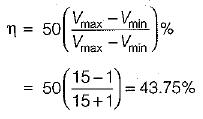Courses

# Large Signal Amplifiers

## 10 Questions MCQ Test Topicwise Question Bank for Electronics Engineering | Large Signal Amplifiers

Description
This mock test of Large Signal Amplifiers for Electronics and Communication Engineering (ECE) helps you for every Electronics and Communication Engineering (ECE) entrance exam. This contains 10 Multiple Choice Questions for Electronics and Communication Engineering (ECE) Large Signal Amplifiers (mcq) to study with solutions a complete question bank. The solved questions answers in this Large Signal Amplifiers quiz give you a good mix of easy questions and tough questions. Electronics and Communication Engineering (ECE) students definitely take this Large Signal Amplifiers exercise for a better result in the exam. You can find other Large Signal Amplifiers extra questions, long questions & short questions for Electronics and Communication Engineering (ECE) on EduRev as well by searching above.
QUESTION: 1

Solution:
QUESTION: 2

### A common-emitter amplifier, with a self-bias circuit to bias the transistor in the active mode, -is an example of a class ____ amplifier.

Solution:

The O-point of class-A amplifier is located in active region i.e. at the mid-point of dc load line as shown below.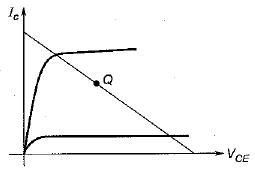QUESTION: 3

### The advantages of class B as compared with class A operation are 1. efficiency is higher. 2. possible to obtain greater power output. 3. less distortion and noise interference. 4. negligible power loss at no input signal. Of these the statements:

Solution:

Compared to class A operation, class B operation has

• higher efficiency (r| = 78.5%)
• greater output power
• more distortion and noise interference.
• negligible power loss at no input signal i.e. no power drain since transistor in class B operation will not consume any power if signal is not applied.

Hence, statements 1,2 and 4 are correct.

QUESTION: 4

Assertion (A): In a system where the power supply is limited, such as those operating from solar cells or a battery, the output power is usually delivered through a push-pull class B transistor circuit.

Reason (R): It is possible to obtain greater power output, higher efficiency and negligible power loss at no signal with the help of a push- pull class B transistor circuit.

Solution:
QUESTION: 5

For a given transistor, the thermal resistance is 8 °C/W and the ambient temperature TA is 27°C. if the transistor dissipates 3 W of power, the junction temperature Tj will be

Solution:

We know that,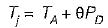or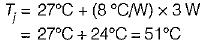QUESTION: 6

Crossover distortion refers to the distortion caused due to

Solution:
QUESTION: 7

In a class B amplifier, VCE(min) = 2 V and supply voltage Vcc = 15 V. The collector circuit efficiency is approximately

Solution:

Collector circuit efficiency is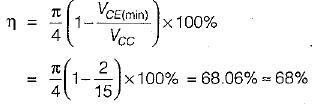QUESTION: 8

The maximum theoretical efficiency of a class A amplifier can be

Solution:
QUESTION: 9

For a transistor, Tj = 160°C, TA = 40°C and θJ-A = 80°C/W. What is the power that the transistor can safely dissipate in free air?

Solution:

The power dissipated by the transistor is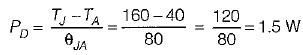QUESTION: 10

ln a class A amplifier, VCE(max) = 15 VCE(min) = 1 V. The overall efficiency for series fed load and transformer coupled load are respectively

Solution:

For series-fed load, overall efficiency,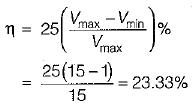For transformer-coupled load, overall efficiency,